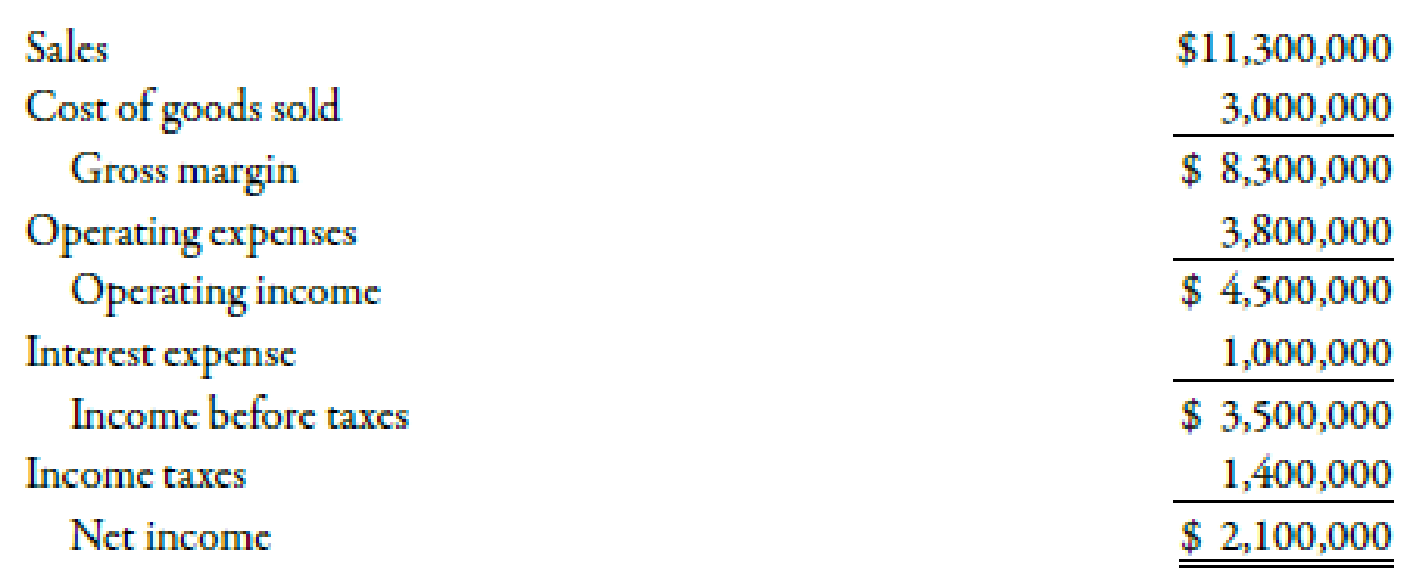# Juroe Company provided the following income statement for last year: Juroe’s balance sheet as of December 31 last year showed total liabilities of $10,250,000, total equity of$6,150,000, and total assets of $16,400,000. Refer to the information for Juroe Company on the previous page. Also, assume that Juroe’s total assets at the beginning of last year equaled$17,350,000 and that the tax rate applicable to Juroe is 40%. Required: Note: Round answers to two decimal places. 1. Calculate the average total assets. 2. Calculate the return on assets.### Managerial Accounting: The Corners...

7th Edition
Maryanne M. Mowen + 2 others
Publisher: Cengage Learning
ISBN: 9781337115773

#### Solutions

Chapter
Section### Managerial Accounting: The Corners...

7th Edition
Maryanne M. Mowen + 2 others
Publisher: Cengage Learning
ISBN: 9781337115773
Chapter 15, Problem 52E
Textbook Problem
46 views

## Juroe Company provided the following income statement for last year:Juroe’s balance sheet as of December 31 last year showed total liabilities of $10,250,000, total equity of$6,150,000, and total assets of $16,400,000.Refer to the information for Juroe Company on the previous page. Also, assume that Juroe’s total assets at the beginning of last year equaled$17,350,000 and that the tax rate applicable to Juroe is 40%.Required:Note: Round answers to two decimal places. 1. Calculate the average total assets. 2. Calculate the return on assets.

1.

To determine

Compute average total assets.

### Explanation of Solution

Return on Assets Ratio

Return on assets evaluates the efficiency of assets by computing return on total assets utilized to produce profits. It is calculated by adding after tax interest expense to net income and divide the result by average total assets.

Use the following formula to compute average assets:

Average assets=Opening assets+closing assets2

2.

To determine

Compute return on assets.

### Still sussing out bartleby?

Check out a sample textbook solution.

See a sample solution

#### The Solution to Your Study Problems

Bartleby provides explanations to thousands of textbook problems written by our experts, many with advanced degrees!

Get Started